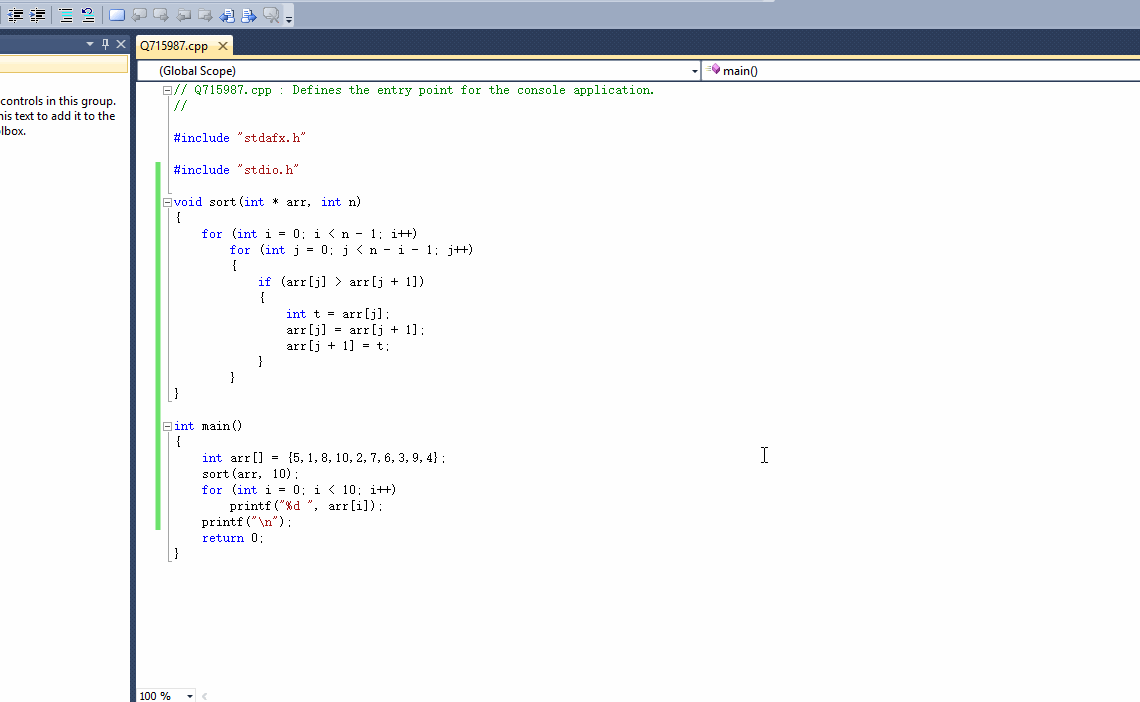1个回答

# 如果问题得到解决，请点我回答左上角的采纳和向上的箭头，谢谢

``````#include "stdio.h"

void sort(int * arr, int n)
{
for (int i = 0; i < n - 1; i++)
for (int j = 0; j < n - i - 1; j++)
{
if (arr[j] > arr[j + 1])
{
int t = arr[j];
arr[j] = arr[j + 1];
arr[j + 1] = t;
}
}
}

int main()
{
int arr[] = {5,1,8,10,2,7,6,3,9,4};
sort(arr, 10);
for (int i = 0; i < 10; i++)
printf("%d ", arr[i]);
printf("\n");
return 0;
}
``````c语言主函数在调用求平均数的函数后就直接结束了

C中对排序好了后的数组中插入一个数在输出

C语言大佬求助；输入5个学生成绩，写一个函数，当主函数调用此函数后，能求出平均分、最高分和最低分

bool imageSubtract(IplImage* src, IplImage* dst) { int row = 0;//行 int col = 0;//列 uchar* pSrcData = NULL; uchar* pDstData = NULL; int stepSrc = 0; int stepDst = 0; if (!src || !dst) { return 0; } if ((src->height != dst->height) && (src->width != dst->width)) { return 0; } pSrcData = (uchar *)src->imageData; stepSrc = src->widthStep / sizeof(uchar); pDstData = (uchar *)src->imageData; stepDst = dst->widthStep / sizeof(uchar); for (row = 0;row <src->height;row++) { for (col = 0;col<src->width;col++) { pDstData[row*stepDst + col] = abs(pSrcData[row*stepSrc + col] - pDstData[row*stepDst + col]); } } return 1; }

C语言编程 作业求助 求大神来帮忙

1、写一个函数计算1+4+9+16+25+...+10000的值。 2、写一个函数，计算1-2+3-4+5-6+...-10000的值。 3、写一个函数，判断一个四位数是否符合如下条件：这个四位数的逆序排列所得的四位数是它自身的整数倍，如果符合，则返回1，不符合返回0。在主程序中调用这个函数，输出符合这个条件的所有的四位数。 4、写一个函数，输出如下格式的图形，要求输出的行数由参数决定，在主函数中输入行数，调用子函数，输出相应行数的图形： * *** ****** ******** ****** *** * 5、如果有一个正整数从左、右来读都是一样的，则称为回文式数(简称回数)；比如101，32123，999都是回数。数学中有名的“回数猜想”之迷，至今未解决。 回数猜想： 任取一个数，再把它倒过来，并把这两个数相加，然后把这个和数再倒过来，与原和数相加，重复此过程，一定能获得一个回数。 例： 68 倒过来是 86， 68+86= 154，154+541= 605，605+506=1111 (回数) 编程，输入任意整数，按上述方法产生一个回数，为简便起见，最多计算7步，看是否能得到一个回数。 要求： 1）主函数中接收键盘数据，必须用scanf(“%ld”,&变量名)接收整型变量，显示该数与其倒数的和，输出每一步计算步骤。 2）子函数1，计算该数的倒数。 3）子函数2，验证和是否为回数。 4）如果经过7次以内的计算得出的数是回数，则主函数打印“经过n次计算，得到回数”，超过7次未能得到回数，显示，“经过n次计算，未得到回数”。

C语言，请问怎么调用这个求平均值的函数?

int main(int argc, char const *argv[]) { int a = {1, 2, 3, 4, 5}; int i; recui(a, 5); for(i=0; i<5; i++) { printf("%d ", a[i]); } printf("\n"); return 0; } void recui(int a[], int k) { int tmp; if()// 填空 { recui();//填空 tmp = a; a = a[k - 1]; a[k - 1] = tmp; } } 应该怎么填呢？

C语言的编程，输入一个英文句子，将每个单词的第一个字母改成大写字母

Problem Description 输入一个英文句子，将每个单词的第一个字母改成大写字母。 Input 输入数据包含多个测试实例，每个测试实例是一个长度不超过100的英文句子，占一行。 Output 请输出按照要求改写后的英文句子。 Sample Input i like acm i want to get an accepted Sample Output I Like Acm I Want To Get An Accepted

c++编写学生信息管理系统，请大神看看我的程序，怎么解决异常。

#include<iostream> #include<fstream> #include<string> #include<stdio.h> #include<stdlib.h> #include<conio.h> //#include<iomanip> using namespace std; #define OK 1 #define ERROR 0 #define OVERFLOW -2 #define LEN(a) sizeof(a)/sizeof(a) typedef int Status; //Status 是函数返回值类型，其值是函数结果状态代码。 typedef int ElemType; //ElemType 为可定义的数据类型，此设为int类型 #define MAXSIZE 100//顺序表可能达到的最大长度 //学生信息的定义： typedef struct { char no; //8位学号 char name; //姓名 int age;//年龄 int sex; //性别 ElemType price; //成绩 }Student; //顺序表的定义（第一个程序用） typedef struct { Student *elem; //指向数据元素的基地址 int length; //线性表的当前长度 }SqList; int u=0,a=0,b=0,c=0; int i; //初始化顺序表 Status InitList(SqList &L) //构造一个空的顺序表L { //算法2.1 顺序表的初始化 L.elem = new Student[MAXSIZE]; //为顺序表分配一个大小为MAXSIZE的数组空间 if (!L.elem) exit(OVERFLOW); //存储分配失败退出 L.length = 0; //空表长度为0 return OK; } //从文件中读取数据创建顺序表 Status CreatList(SqList &L){ fstream file("student.txt"); //打开文件进行读写操作 const int Line_Length=100; char str[Line_Length]; //file.open("student.txt"); if (!file) { cout << "未找到相关文件，无法打开！" << endl; exit(ERROR); } //将文件信息读入顺序表 while(file.getline(str,Line_Length)) { cout<<"Read form student.txt:"<<str<<endl; } file.close(); return OK; } //逐个显示表中学生信息 void show(SqList &L) { cout<<"学生信息如下："<<endl; for(int i=1;i<=L.length;i++){ cout<<"学号"<<L.elem[i].no<<" "<<"姓名："<<L.elem[i].name<<" " <<"性别："<<L.elem[i].sex<<" "<<"年龄："<<L.elem[i].age<<" " <<"成绩："<<L.elem[i].price<<L.elem[i].price <<L.elem[i].price<<L.elem[i].price<<endl; } } //将学生信息插入表中指定位置 void ListInsert(SqList &L){ int i; cout<<"请输入要插入的位置："<<endl; cin>>i; if(L.length==MAXSIZE) cout<<"没有内存空间！"<<endl; else if(i<1||i>L.length+1) cout<<"输入位置不正确，请检查！"<<endl; else{ for(int j=L.length;j>=i;j--) L.elem[j+1]=L.elem[j]; cout<<"请分别输入要插入学生的学号，姓名，性别，年龄，四科成绩："<<endl; cout<<"学生学号："; cin>>L.elem[i].no; cout<<"学生姓名："; cin>>L.elem[i].name; cout<<"学生性别："; cin>>L.elem[i].sex; cout<<"学生年龄："; cin>>L.elem[i].age; cout<<"学生成绩："; cin>>L.elem[i].price; cin>>L.elem[i].price; cin>>L.elem[i].price; cin>>L.elem[i].price; cout<<endl; cout<<"插入成功！"<<endl; cout<<"插入的学生信息为："<<endl; cout<<"学号："<<L.elem[i].no<<endl; cout<<"姓名："<<L.elem[i].name<<endl; cout<<"性别："<<L.elem[i].sex<<endl; cout<<"年龄："<<L.elem[i].age<<endl; cout<<"成绩："<<L.elem[i].price<<L.elem[i].price <<L.elem[i].price<<L.elem[i].price<<endl; } } //删除指定学生的信息 Status ListDelete(SqList &L,int i) { FILE *fp; if(i>=0&&i<=L.length) { for(int j=i;j<L.length;j++) { //将指定位置之后的元素依次往前移 L.elem[j-1] =L.elem[j]; } --L.length; } else{ printf("输入的位置有误！"); } fp=fopen("Database.txt","w"); if(fp==NULL) { printf("文件无法打开!\n"); exit(ERROR); } return OK; } //统计表中学生个数 int GetLength(SqList L){ return L.length; } //直接排序 void InsertSort(SqList &L){ for(int i=2;i<=L.length;i++){ if(strcmp(L.elem[i].name,L.elem[i-1].name)<0) { L.elem=L.elem[i]; L.elem[i]=L.elem[i-1]; for(int j=i-2;strcmp(L.elem.name,L.elem[j].name)<0;j--) { L.elem[j+1]=L.elem[j]; L.elem[j]=L.elem; } } } a=1; } //快速排序 int Partition(SqList &L,int low,int high) { char pivotkey; //设置关键字pivotkey L.elem=L.elem[low];// 把表的第一个记录作为枢轴 strcpy(pivotkey,L.elem[low].no);//将学号作为关键字放入pivotkey中保存 while(low< high) { while((low<high)&&(L.elem[high].no>=pivotkey)) --high; L.elem[low]=L.elem[high]; while((low<high)&&(L.elem[low].no<=pivotkey)) ++low; L.elem[high]=L.elem[low]; } L.elem[low]=L.elem; return low; } void QSort(SqList &L,int low,int high) { if(low<high) { int pivotloc=Partition(L,low,high); QSort(L,low,pivotloc-1); //对低子表递归排序，pivotloc是枢轴位置 QSort(L,pivotloc+1,high); //对高子表递归排序 } } void QuickSort(SqList &L) { QSort(L,1,L.length); } //根据姓名进行折半查找，使用递归，返回学生学号和成绩。 int Search_Dname(SqList &L,char a[],int low,int high) { low=1; high=L.length; int mid=(low+high)/2; if(strcmp(a,L.elem[mid].name)==0) return mid; if(strcmp(a,L.elem[mid].name)<0) return Search_Dname(L,a,low,mid-1); if(strcmp(a,L.elem[mid].name)>0) return Search_Dname(L,a,mid+1,high); return 0; } //根据姓名进行折半查找，不使用递归，返回学生的学号和成绩。 int Search_No(SqList &L,char b[]) { int mid; int low=1; int high=L.length; while(low<=high){ mid=(high+low)/2; if(strcmp(b,L.elem[mid].name)==0) return mid; else if(strcmp(b,L.elem[mid].name)<0) high=mid-1; else low=mid+1; } return 0; } void stop(){ char i; cout<<"您真的要退出程序吗 ？"<<endl; cout<<"1"<<"确定"; cout<<"2"<<"取消"; cin>>i; switch(i){ case '1':cout<<"谢谢使用！"<<endl;exit(0); case '2':break; default: cout<<"输入错误！"<<endl; cin>>i;break; } } //主函数 void main(){ //freopen("student.txt","r","stdin"); //freopen("student2.txt","w",stdout); //int no; int n; SqList L; while(1){ cout<<"*************欢迎使用学生信息查询系统******************"<<endl; cout<<"1.初始化顺序表。"<<endl; cout<<"2.从文件中读取创建顺序表。"<<endl; cout<<"3.逐个显示学生相关信息。"<<endl; cout<<"4.插入学生信息到指定位置。"<<endl; cout<<"5.删除指定位置学生。"<<endl; cout<<"6.统计表中学生人数。"<<endl; cout<<"7.直接插入排序按学生姓名排序。"<<endl; cout<<"8.快速排序按学生学号排序。"<<endl; cout<<"9.根据姓名进行折半查找（递归）。"<<endl; cout<<"10.跟学号进行折半查找（非递归）。"<<endl; cout<<"0.退出系统。"<<endl; cout<<endl;//设置菜单栏 cout<<"请选择您需要的服务："; cin>>n; switch(n) { case 1: if (InitList(L)) cout << "成功建立顺序表\n\n"; else cout << "顺序表建立失败\n\n"; break; case 2: CreatList(L); cout<<"读入文件成功！"<<endl;break; case 3: if(u){ show(L); getch(); } case 4: if(u){ ListInsert(L); getch(); }else cout<<"请先读入文件！"<<endl;break; case 5: if(u){ int i; ListDelete(L,i); getch(); }else cout<<"请先读入文件！"<<endl;break; case 6: { if(u){ int i=GetLength(L); cout<<"学生总人数为："<<i<<"个"<<endl;break; } case 7: if(u){ InsertSort(L); b=0; cout<<"直接插入排序成功，按姓名从小到大排序为："<<endl; for(int k=1;k<=L.length;k++){ cout<<"学号"<<L.elem[i].no<<" "<<"姓名："<<L.elem[i].name<<" " <<"性别："<<L.elem[i].sex<<" "<<"年龄："<<L.elem[i].age<<" " <<"成绩："<<L.elem[i].price<<L.elem[i].price <<L.elem[i].price<<L.elem[i].price<<endl; getch(); } } else cout<<"请先读入文件！"<<endl;break; } case 8: if(u){ QSort(L,1,L.length); a=1; cout<<"快速排序成功，按学号从小到大输出如下："<<endl; for(int k=1;k<=L.length;k++){ cout<<"学号"<<L.elem[i].no<<" "<<"姓名："<<L.elem[i].name<<" " <<"性别："<<L.elem[i].sex<<" "<<"年龄："<<L.elem[i].age<<" " <<"成绩："<<L.elem[i].price<<L.elem[i].price <<L.elem[i].price<<L.elem[i].price<<endl; } getch(); }else cout<<"请先读入文件！"<<endl;break; case 9: if(u==0) cout<<"请先读入文件！"<<endl; else if(u==1&&a==0) cout<<"请先使用命令6进行排序。"<<endl; else{ char a; cout<<"请输入你要查找的学生名字："; cin>>a; int i; i=Search_Dname(L,a,0,L.length); if(i>=0) cout<<"您查找的学生个人信息为：学号"<<L.elem[i].no<<" "<<"姓名："<<L.elem[i].name<<" " <<"性别："<<L.elem[i].sex<<" "<<"年龄："<<L.elem[i].age<<" " <<"成绩："<<L.elem[i].price<<L.elem[i].price <<L.elem[i].price<<L.elem[i].price<<endl; else cout<<"学生不存在！"<<endl; getch(); }break; case 10: if(u==0) cout<<"请先读入文件！"<<endl; else if(u==1&&a==0) cout<<"请先使用命令7进行排序。"<<endl; else{ char b; cout<<"请输入您要查找的学生学号："; cin>>b; int j=Search_No(L,b); if(j>=0) cout<<"您查找的学生个人信息为：学号"<<L.elem[i].no<<" "<<"姓名："<<L.elem[i].name<<" " <<"性别："<<L.elem[i].sex<<" "<<"年龄："<<L.elem[i].age<<" " <<"成绩："<<L.elem[i].price<<L.elem[i].price <<L.elem[i].price<<L.elem[i].price<<endl; else cout<<"学生不存在！"<<endl; getch(); }break; case 0:cout<<endl; stop();break; default: cout<<"输出错误！"<<endl;break; } cout<<endl; } }

Java校招入职华为，半年后我跑路了

Java基础知识面试题（2020最新版）

@程序员：GitHub这个项目快薅羊毛

loonggg读完需要3分钟速读仅需 1 分钟大家好，我是你们的校长。我之前讲过，这年头，只要肯动脑，肯行动，程序员凭借自己的技术，赚钱的方式还是有很多种的。仅仅靠在公司出卖自己的劳动时...

win10暴力查看wifi密码

MySQL数据库面试题（2020最新版）

2020阿里全球数学大赛：3万名高手、4道题、2天2夜未交卷

HTTP与HTTPS的区别

HashMap底层实现原理，红黑树，B+树，B树的结构原理 Spring的AOP和IOC是什么？它们常见的使用场景有哪些？Spring事务，事务的属性，传播行为，数据库隔离级别 Spring和SpringMVC，MyBatis以及SpringBoot的注解分别有哪些？SpringMVC的工作原理，SpringBoot框架的优点，MyBatis框架的优点 SpringCould组件有哪些，他们...